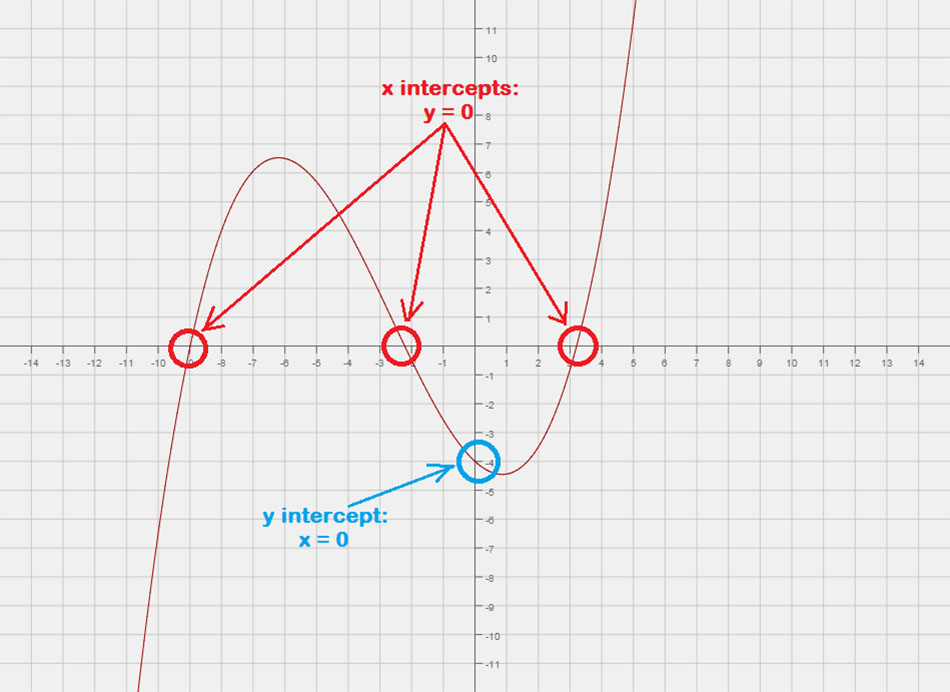## How to find the x intercepts and y intercepts of an equation

Question

Last Updated on May 15, 2020 by Ephraim Iyodo

It’s very simple to identify the x and y intercepts on a graph, but students frequently struggle to find them using only the equation. However, it just requires a simple trick:

To find the x intercept(s) of an equation, substitute in y = 0 and solve for x.

To find the y intercept(s) of an equation, substitute in x = 0 and solve for y.# The X-Intercepts

The x-intercepts are points where the graph of a function or an equation crosses or “touches” the x-axis of the Cartesian Plane. You may think of this as a point with y-value of zero.

• To find the x-intercepts of an equation, let y = 0 then solve for x.
• In a point notation, it is written as \left( {x,0} \right).

### x-intercept of a Linear Function or a Straight Line### x-intercepts of a Quadratic Function or Parabola## The Y-Intercepts

The y-intercepts are points where the graph of a function or an equation crosses or “touches” the y-axis of the Cartesian Plane. You may think of this as a point with x-value of zero.

• To find the y-intercepts of an equation, let x = 0 then solve for y.
• In a point notation, it is written as \left( {0,y} \right).

### y-intercept of a Linear Function or a Straight Line### y-intercept of a Quadratic Function or Parabola## Examples of How to Find the x and y-intercepts of a Line, Parabola, and Circle

Example 1: From the graph, describe the x and y-intercepts using point notation.

The graph crosses the x-axis at = 1 and = 3, therefore, we can write the x-intercepts as points (1,0) and (–3, 0).

Similarly, the graph crosses the y-axis at = 3. Its y-intercept can be written as the point (0,3).

Example 2: Find the x and y-intercepts of the line y = –2+ 4.

To find the x-intercepts algebraically, we let y = 0 in the equation and then solve for values of x. In the same manner, to find for y-intercepts algebraically, we let = 0 in the equation and then solve for y.

Here’s the graph to verify that our answers are correct.

Example 3: Find the x and y-intercepts of the quadratic equation y = x2 − 2x − 3.

The graph of this quadratic equation is a parabola. We expect it to have a “U” shape where it would either open up or down.

To solve for the x-intercept of this problem, you will factor a simple trinomial. Then you set each binomial factor equal to zero and solve for x.

Our solved values for both x and y-intercepts match with the graphical solution.

Example 4: Find the x and y-intercepts of the quadratic equation y = 3x2 + 1.

This is an example where the graph of the equation has a y-intercept but without an x-intercept.

• Let’s find the y-intercept first because it’s extremely easy! Plug in x = 0 then solve for y.
• Now for the x-intercept. Plug in y = 0, and solve for x.

The square root of a negative number is imaginary. This suggests that this equation does not have an x-intercept!

The graph can verify what’s going on. Notice that the graph crossed the y-axis at (0,1), but never did with the x-axis.

Example 5: Find the x and y intercepts of the circle (x + 4)2 + (y + 2)2 = 8.

This is a good example to illustrate that it is possible for the graph of an equation to have x-intercepts but without y-intercepts.

When solving for y, we arrived at the situation of trying to get the square root of a negative number. The answer is imaginary, thus, no solution. That means the equation doesn’t have any y-intercepts.

The graph verifies that we are right for the values of our x-intercepts, and it has no y-intercepts.

Credit:

https://www.studiosity.com/blog/

https://www.chilimath.com/lessons/intermediate-algebra/finding-x-y-intercepts/

0
2 years 0 Answers 3364 views 0
Brilliantly Safe & Student-Centered Learning Platform 2021